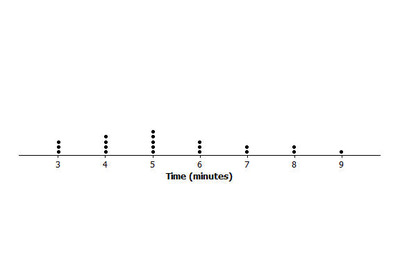# Puzzle Times

Alignments to Content Standards: 6.SP.B.4 6.SP.B.5.c

Each of the 20 students in Mr. Anderson's class timed how long it took them to solve a puzzle. Their times (in minutes) are listed below:

 Student Time (minutes) 1 2 3 4 5 6 7 8 9 10 11 12 13 14 15 16 17 18 19 20 3 5 4 6 4 8 5 4 9 5 3 4 7 5 8 6 3 6 5 7
1. Display the data using a dot plot.
2. Find the mean and median of the data. Does it surprise you that the values of the mean and median are not equal? Explain why or why not.

## IM Commentary

This is a simple task designed to assess students’ ability to construct a dot plot (6.SP.4) and to calculate and compare measures of center (6.SP.5.c).

It also provides an opportunity to discus how the relative values of the mean and the median relate to the shape of a data distribution. Here, the data distribution is a bit skewed to the right, so the value of the mean is greater than the value of the median. But, since the distribution is not far from symmetric, the value of the mean and the value of the median are close.

## Solution

1. A dot plot showing the times is shown here:In both cases we can see that the most common times were 3, 4, 5, and 6 minutes, although there are also several times of 7, 8, and 9 minutes. There were no times of 1, 2, or 10 minutes.

2. To find the mean, we need to add up all of the times and divide by the number of data points, 20. This gives $$\frac{3 \times 3 + 4 \times 4 + 5 \times 5 + 3 \times 6 + 2\times 7 + 2 \times 8 + 1 \times 9}{20}.$$ Evaluating this expression gives 5.35 minutes.

There are 20 numbers in the list so the median will be average of the middle two numbers: $$3, 3, 3, 4, 4, 4, 4, 5, 5, {\bf {\large 5}}, {\bf {\large 5}}, 5, 6, 6, 6, 7, 7, 8, 8, 9.$$ The middle two numbers are both 5's so the median is 5 minutes.

These two results make sense because the data are slightly skewed toward the larger numbers. Since the median is 5 we expect the mean to be larger than 5.